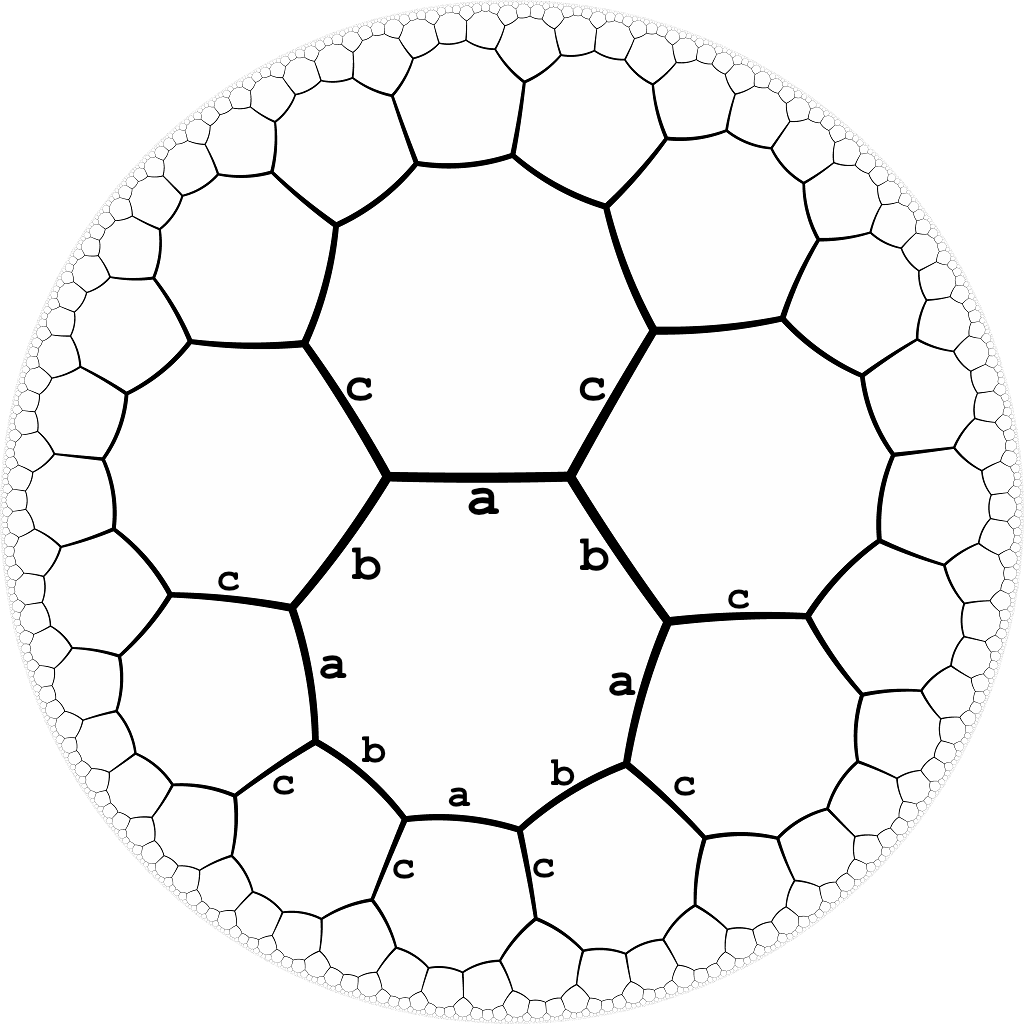시간 제한 메모리 제한 제출 정답 맞은 사람 정답 비율
1 초 256 MB 1 1 1 100.000%

## 문제

Below is a picture of an infinite hyperbolic tessellation of octagons. If we think of this as a graph of vertices (of degree three), then there exists an isomorphism of the graph which maps any vertex $x$ onto any other vertex $y$.  Every edge is given a label from the set {a, b, c} in such a way that every vertex has all three types of edges incident on it, and the labels alternate around each octagon. Part of this labeling is illustrated in the diagram below.So a path in this graph (starting from any vertex) can be specified by a sequence of edge labels. Your job is to write a program which, given a sequence of labels such as "abcbcbcabcaccadb", returns "closed" if the path ends on the same vertex where it starts, and returns "open" otherwise.

## 입력

The input is a string of length at least $1$ and at most $100\,000$ consisting of letters "a", "b" and "c".

## 출력

The output should be one line with one word: either "closed" or "open".

## 예제 입력 1

abababab


## 예제 출력 1

closed


## 예제 입력 2

abcbcbcbcba


## 예제 출력 2

open